Co-efficient of variation

Chapter 13 Class 11 Statistics
Concept wise

Here, we list all Statistics Formulas for your use.

The formula list includes

## Range

Range = Maximum value – Minimum Value

For data

5, 10, 15, 18, 21, 24, 24, 13, 2, 9, 9, 18, 18, 32, 28, 3, 14, 25, 3, 27

Maximum value = 32

Minimum value = 2

Thus,

Range = 32 – 2 = 30

## Mean of Raw, Discrete and Grouped Data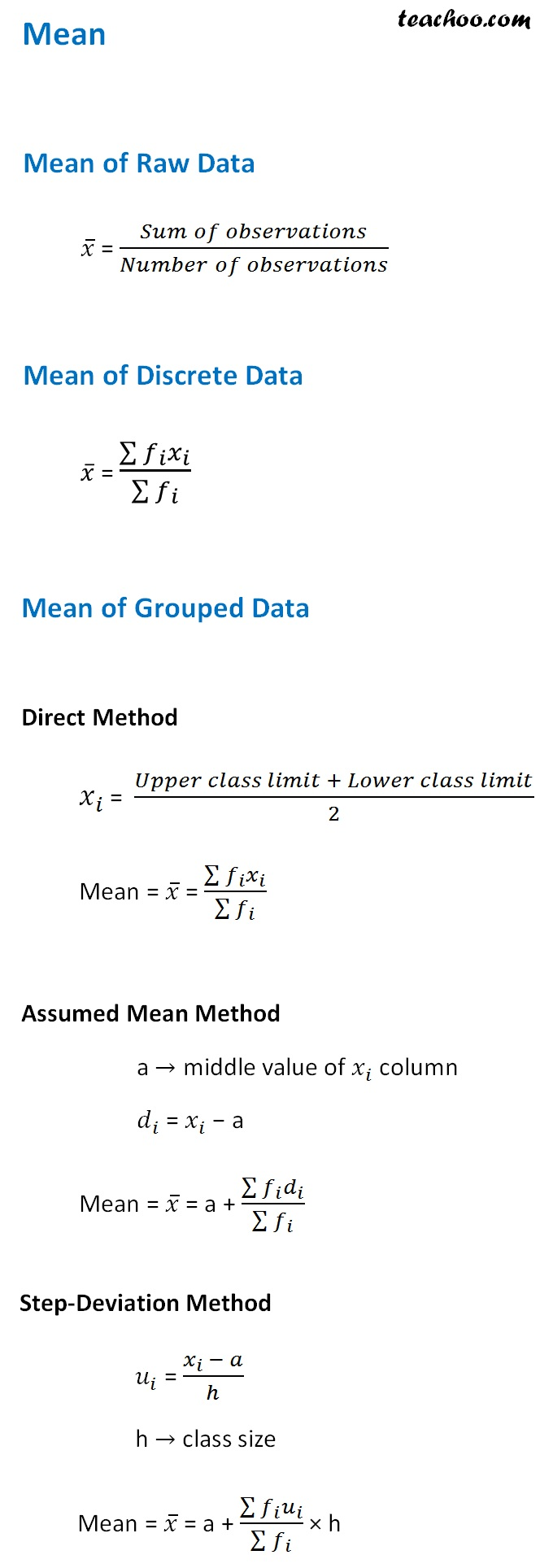## Median of Raw, Discrete and Grouped Data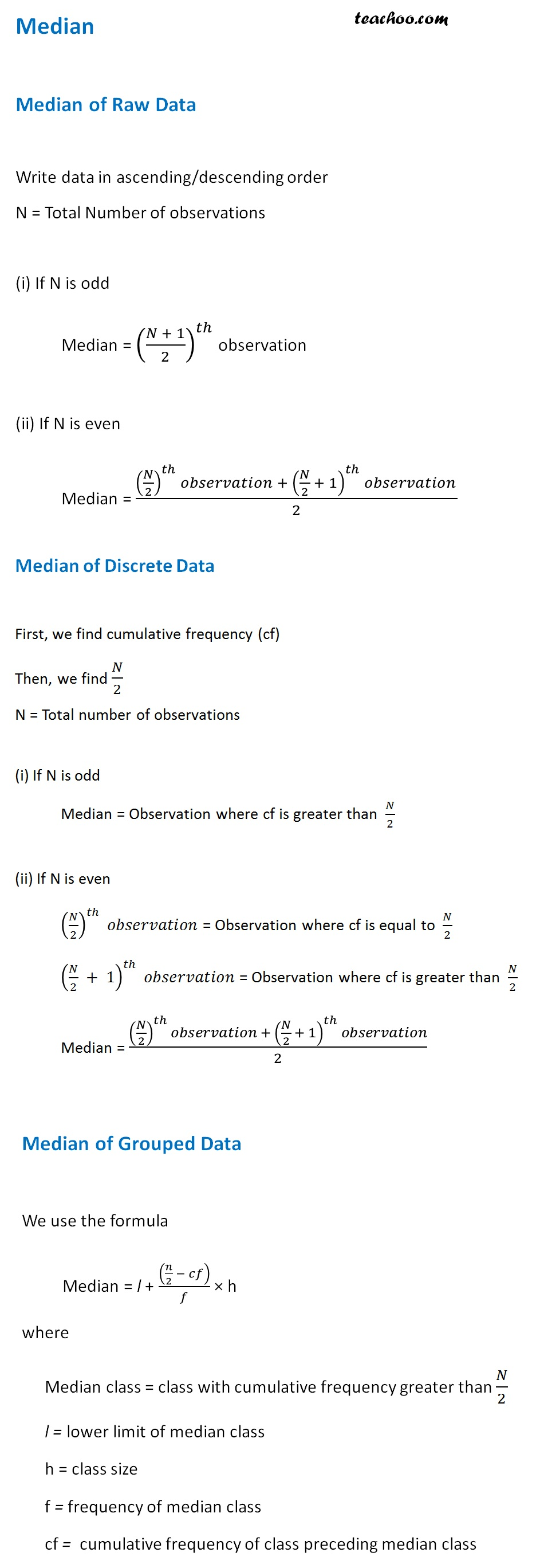We can also find median using Ogive curve

We draw less than and more than ogive,

and their intersection is the median

## Mode of Raw, Discrete and Grouped Data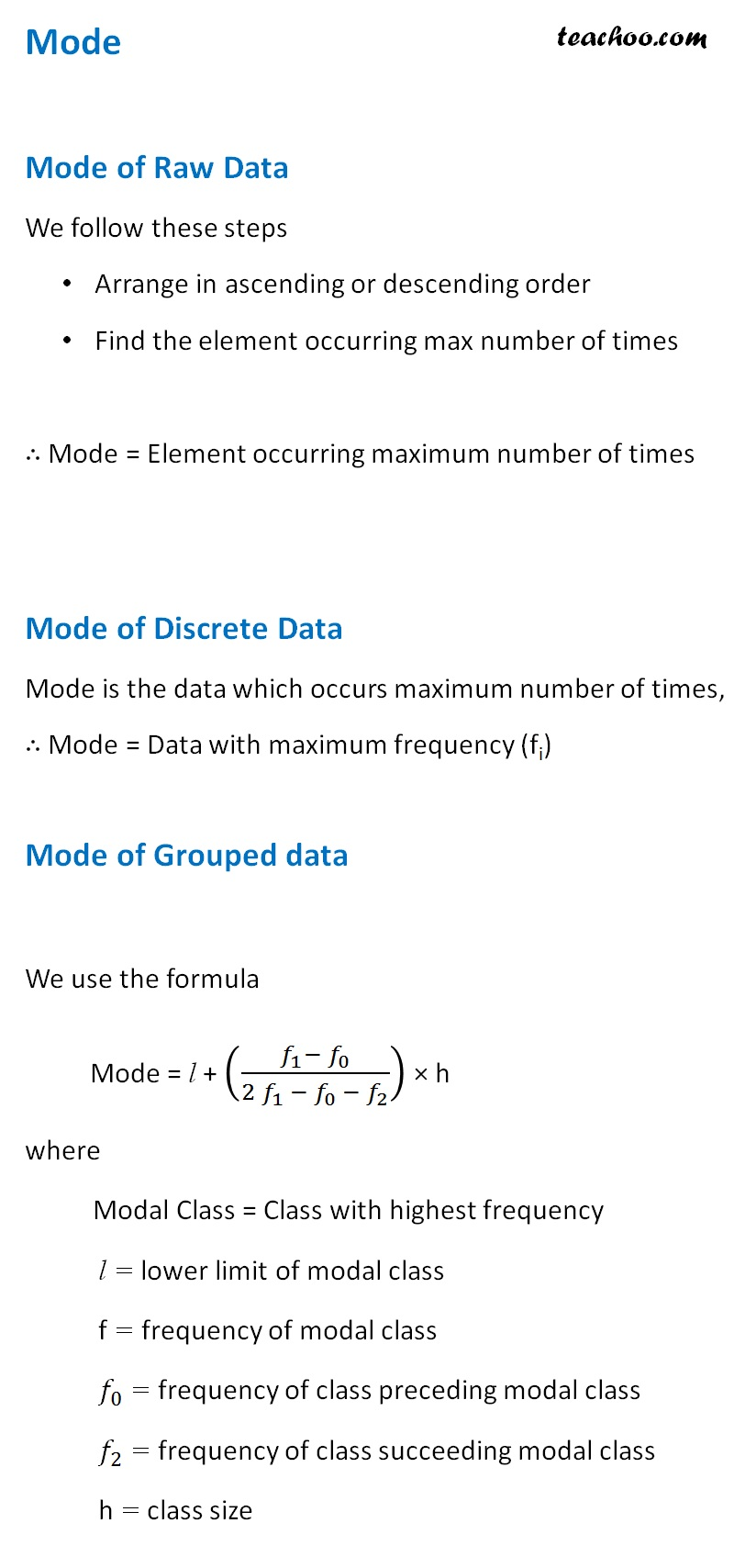## Mean deviation about Mean and Median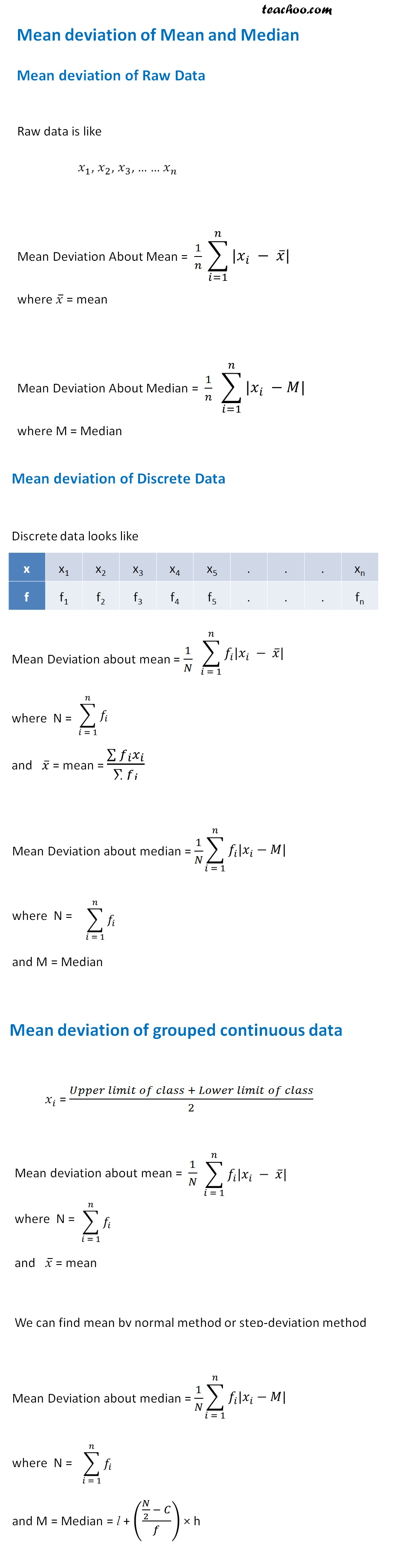## Variance and Standard Deviation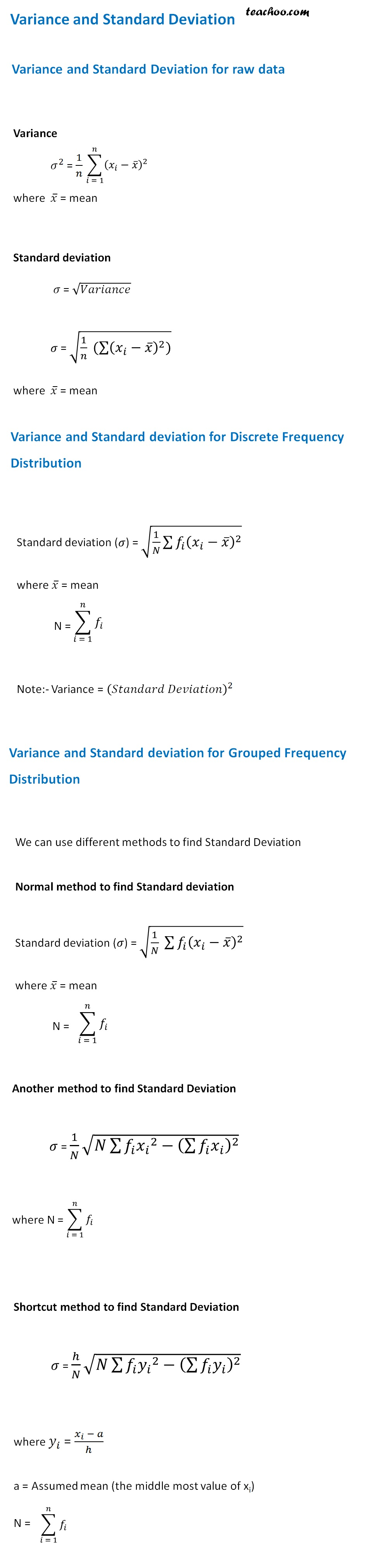## Coefficient of Variation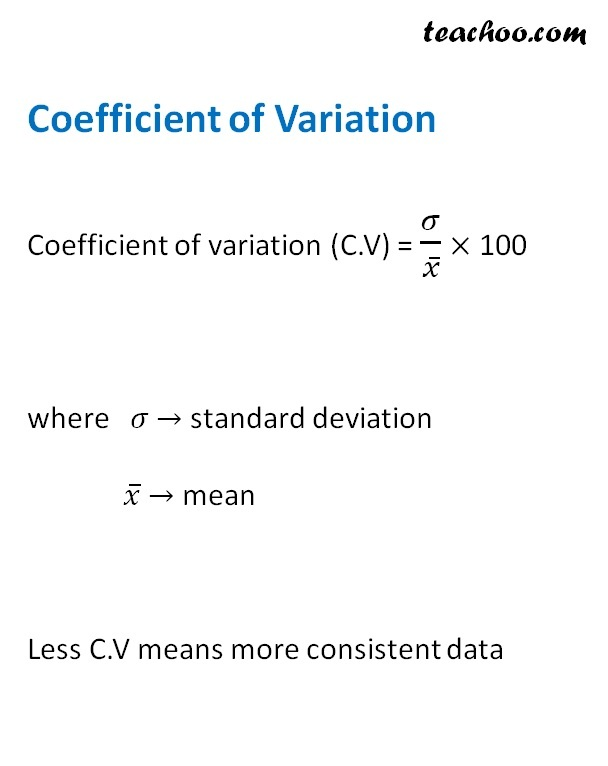Learn in your speed, with individual attention - Teachoo Maths 1-on-1 Class

### Transcript

Mean of Raw Data 𝑥 ̅ = (𝑆𝑢𝑚 𝑜𝑓 𝑜𝑏𝑠𝑒𝑟𝑣𝑎𝑡𝑖𝑜𝑛𝑠)/(𝑁𝑢𝑚𝑏𝑒𝑟 𝑜𝑓 𝑜𝑏𝑠𝑒𝑟𝑣𝑎𝑡𝑖𝑜𝑛𝑠) Mean of Discrete Data 𝑥 ̅ = (∑▒〖𝑓_𝑖 𝑥_𝑖 〗)/(∑▒𝑓_𝑖 ) Mean of Grouped Data Direct Method 𝑥_𝑖 = (𝑈𝑝𝑝𝑒𝑟 𝑐𝑙𝑎𝑠𝑠 𝑙𝑖𝑚𝑖𝑡 + 𝐿𝑜𝑤𝑒𝑟 𝑐𝑙𝑎𝑠𝑠 𝑙𝑖𝑚𝑖𝑡)/2 Mean = 𝑥 ̅ = (∑▒〖𝑓_𝑖 𝑥_𝑖 〗)/(∑▒𝑓_𝑖 ) Assumed Mean Method a → middle value of 𝑥_𝑖 column 𝑑_𝑖 = 𝑥_𝑖 − a Mean = 𝑥 ̅ = a + (∑▒〖𝑓_𝑖 𝑑_𝑖 〗)/(∑▒𝑓_𝑖 ) Step-Deviation Method 𝑢_𝑖 = (𝑥_𝑖 − 𝑎)/ℎ h → class size Mean = 𝑥 ̅ = a + (∑▒〖𝑓_𝑖 𝑢_𝑖 〗)/(∑▒𝑓_𝑖 ) × h Median Median of Raw Data Write data in ascending/descending order N = Total Number of observations (i) If N is odd Median = ((𝑁 + 1)/2)^𝑡ℎ observation (ii) If N is even Median = ((𝑁/2)^𝑡ℎ 𝑜𝑏𝑠𝑒𝑟𝑣𝑎𝑡𝑖𝑜𝑛 + (𝑁/2 + 1)^𝑡ℎ 𝑜𝑏𝑠𝑒𝑟𝑣𝑎𝑡𝑖𝑜𝑛)/2 Median of Discrete Data First, we find cumulative frequency (cf) Then, we find 𝑁/2 N = Total number of observations (i) If N is odd Median = Observation where cf is greater than 𝑁/2 (ii) If N is even (𝑁/2)^𝑡ℎ 𝑜𝑏𝑠𝑒𝑟𝑣𝑎𝑡𝑖𝑜𝑛 = Observation where cf is equal to 𝑁/2 (𝑁/2 + 1)^𝑡ℎ 𝑜𝑏𝑠𝑒𝑟𝑣𝑎𝑡𝑖𝑜𝑛 = Observation where cf is greater than 𝑁/2 Median = ((𝑁/2)^𝑡ℎ 𝑜𝑏𝑠𝑒𝑟𝑣𝑎𝑡𝑖𝑜𝑛 + (𝑁/2 + 1)^𝑡ℎ 𝑜𝑏𝑠𝑒𝑟𝑣𝑎𝑡𝑖𝑜𝑛)/2 Median of Grouped Data We use the formula Median = l + ((𝑛/2 − 𝑐𝑓))/𝑓 × h where Median class = class with cumulative frequency greater than 𝑁/2 l = lower limit of median class h = class size f = frequency of median class cf = cumulative frequency of class preceding median class Mode Mode of Raw Data We follow these steps Arrange in ascending or descending order Find the element occurring max number of times ∴ Mode = Element occurring maximum number of times Mode of Discrete Data Mode is the data which occurs maximum number of times, ∴ Mode = Data with maximum frequency (fi) Mode of Grouped data We use the formula Mode = l + ((𝑓_1− 𝑓_0)/(2 𝑓_1 − 𝑓_(0 )− 𝑓_2 )) × h where Modal Class = Class with highest frequency l = lower limit of modal class f = frequency of modal class 𝑓_0 = frequency of class preceding modal class 𝑓_2 = frequency of class succeeding modal class h = class size Mean deviation of Raw Data Raw data is like 𝑥_1, 𝑥_2, 𝑥_3, … … 𝑥_𝑛 Mean Deviation About Mean = 1/𝑛 ∑_(𝑖=1)^𝑛▒〖|𝑥_𝑖 − 𝑥 ̅|〗 where 𝑥 ̅ = mean Mean Deviation About Median = 1/𝑛 ∑_(𝑖=1)^𝑛▒〖|𝑥_𝑖 −𝑀|〗 where M = Median Mean deviation of Discrete Data Discrete data looks like x x1 x2 x3 x4 x5 . . . xn f f1 f2 f3 f4 f5 . . . fn Mean Deviation about mean = 1/𝑁 ∑_(𝑖 = 1)^𝑛▒〖𝑓_𝑖 |𝑥_𝑖 − 𝑥 ̅|〗 where N = ∑_(𝑖 = 1)^𝑛▒𝑓_𝑖 and 𝑥 ̅ = mean = (∑▒〖𝑓_𝑖 𝑥_𝑖 〗)/(∑▒𝑓_𝑖 ) Mean Deviation about median = 1/𝑁 ∑_(𝑖 = 1)^𝑛▒〖𝑓_𝑖 |𝑥_𝑖−𝑀|〗 where N = ∑_(𝑖 = 1)^𝑛▒𝑓_𝑖 and M = Median Mean deviation of grouped continuous data Mean Deviation About Mean 𝑥_𝑖 = (𝑈𝑝𝑝𝑒𝑟 𝑙𝑖𝑚𝑖𝑡 𝑜𝑓 𝑐𝑙𝑎𝑠𝑠 + 𝐿𝑜𝑤𝑒𝑟 𝑙𝑖𝑚𝑖𝑡 𝑜𝑓 𝑐𝑙𝑎𝑠𝑠)/2 Mean deviation about mean = 1/𝑁 ∑_(𝑖 = 1)^𝑛▒〖𝑓_𝑖 |𝑥_𝑖 − 𝑥 ̅|〗 where N = ∑_(𝑖 = 1)^𝑛▒𝑓_𝑖 and 𝑥 ̅ = mean We can find mean by normal method or step-deviation method Mean Deviation about median = 1/𝑁 ∑_(𝑖 = 1)^𝑛▒〖𝑓_𝑖 |𝑥_𝑖−𝑀|〗 where N = ∑_(𝑖 = 1)^𝑛▒𝑓_𝑖 and M = Median = l + ((𝑁/2 − 𝐶)/𝑓) × h Variance and Standard Deviation for raw data Variance 𝜎^2 = 1/𝑛 ∑_(𝑖 = 1)^𝑛▒(𝑥_𝑖−𝑥 ̅ )^2 where 𝑥 ̅ = mean Standard deviation 𝜎 = √𝑉𝑎𝑟𝑖𝑎𝑛𝑐𝑒 𝜎 = √(1/𝑛 (∑▒(𝑥_𝑖−𝑥 ̅ )^2 )) where 𝑥 ̅ = mean Variance and Standard deviation for Discrete Frequency Distribution Standard deviation (𝜎) = √(1/𝑁 ∑▒〖𝑓_𝑖 (𝑥_𝑖−𝑥 ̅ )〗^2 ) where 𝑥 ̅ = mean N = ∑_(𝑖 = 1)^𝑛▒𝑓_𝑖 Note:- Variance = (𝑆𝑡𝑎𝑛𝑑𝑎𝑟𝑑 𝐷𝑒𝑣𝑖𝑎𝑡𝑖𝑜𝑛)^2 Variance and Standard deviation for Grouped Frequency Distribution We can use different methods to find Standard Deviation Normal method to find Standard deviation Standard deviation (𝜎) = √(1/𝑁 ∑▒〖𝑓_𝑖 (𝑥_𝑖−𝑥 ̅ )^2 〗) where 𝑥 ̅ = mean N = ∑_(𝑖 = 1)^𝑛▒𝑓_𝑖 Another method to find Standard Deviation 𝜎 = 1/𝑁 √(𝑁∑▒〖𝑓_𝑖 〖𝑥_𝑖〗^2 〗−(∑▒〖𝑓_𝑖 𝑥_𝑖 〗)^2 ) where N = ∑_(𝑖 = 1)^𝑛▒𝑓_𝑖 Shortcut method to find Standard Deviation 𝜎 = ℎ/𝑁 √(𝑁∑▒〖𝑓_𝑖 〖𝑦_𝑖〗^2 〗−(∑▒〖𝑓_𝑖 𝑦_𝑖 〗)^2 ) where 𝑦_𝑖 = (𝑥_𝑖 − 𝑎)/ℎ a = Assumed mean (the middle most value of xi) N = ∑_(𝑖 = 1)^𝑛▒𝑓_𝑖 Coefficient of Variation Coefficient of variation (C.V) = 𝜎/𝑥 ̅ × 100 where 𝜎 → standard deviation 𝑥 ̅ → mean Less C.V means more consistent data